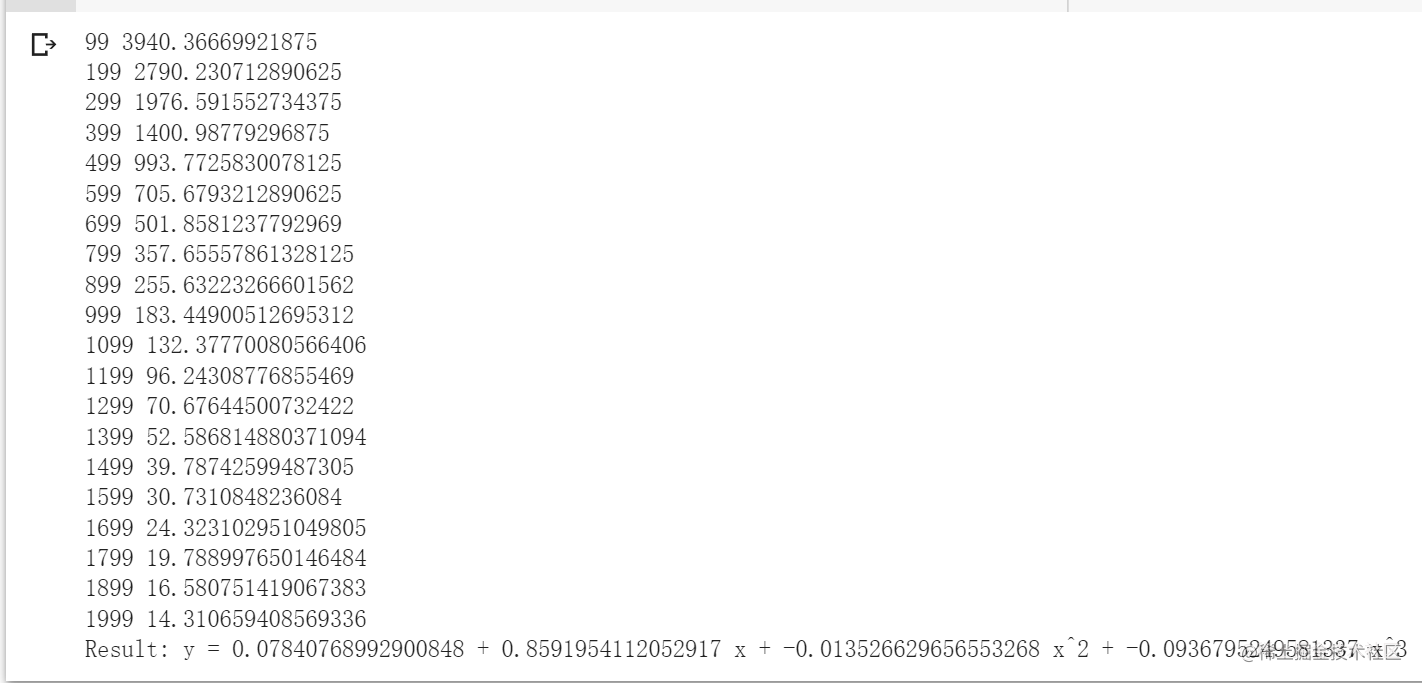## theme: v-green

Pytorch有兩個重要的特徵：

• 使用n維張量進行運算，可以使用GPU加速計算。
• 使用自動微分構建、訓練神經網路

# 從$sin(x)$開始

Tensor和Numpy的用法差不多，但是Tensor可是使用進行加速計算，這比CPU計算要快50倍甚至更多。

py import torch import math

dtype = torch.float device = torch.device("cpu")

# 建立輸入輸出資料，這裡是x和y代表[-π，π]之間的sin(x)的值

x = torch.linspace(-math.pi, math.pi, 2000, device=device, dtype=dtype) y = torch.sin(x)

# 隨機初始化權重

a = torch.randn((), device=device, dtype=dtype) b = torch.randn((), device=device, dtype=dtype) c = torch.randn((), device=device, dtype=dtype) d = torch.randn((), device=device, dtype=dtype)

learning_rate = 1e-6 for t in range(2000): # 前向過程，計算y的預測值 y_pred = a + b * x + c * x 2 + d * x 3

# 計算預測值和真實值的loss
loss = (y_pred - y).pow(2).sum().item()
if t % 100 == 99:
print(t, loss)

# 反向過程計算 a, b, c, d 關於 loss 的梯度
grad_y_pred = 2.0 * (y_pred - y)

# 使用梯度下降更新引數


print(f'Result: y = {a.item()} + {b.item()} x + {c.item()} x^2 + {d.item()} x^3')  結果如下：# 如果換成勒讓德多項式呢？

py import torch import math

dtype = torch.float device = torch.device("cpu")

# 建立輸入輸出資料，這裡是x和y代表[-π，π]之間的sin(x)的值

x = torch.linspace(-math.pi, math.pi, 2000, device=device, dtype=dtype) y = torch.sin(x)

# 隨機初始化權重

a = torch.randn((), device=device, dtype=dtype, requires_grad=True) b = torch.randn((), device=device, dtype=dtype, requires_grad=True) c = torch.randn((), device=device, dtype=dtype, requires_grad=True) d = torch.randn((), device=device, dtype=dtype, requires_grad=True)

learning_rate = 1e-6 for t in range(2000):

# 前向過程，計算y的預測值
y_pred = a + b * x + c * x ** 2 + d * x ** 3

# 計算預測值和真實值的loss
loss = (y_pred - y).pow(2).sum()
if t % 100 == 99:
print(t, loss.item())

loss.backward()

# 使用梯度下降更新引數

# 更新之後將氣度清零，以便下一輪運算，不清零的話它會一直累計


print(f'Result: y = {a.item()} + {b.item()} x + {c.item()} x^2 + {d.item()} x^3')• forward：由輸入張量計算輸出張量
• backward：接收輸出張量相對於某個標量值的梯度，並計算輸入張量相對於相同標量值的梯度。

py import torch import math

    # 在前向過程中我們接受一個輸入張量，並返回一個輸出張量
# ctx是一個上下文物件，用於儲存反向過程的內容
# 你可以用save_for_backward方法快取任意在反向計算過程中要用的物件。

ctx.save_for_backward(input)
return 0.5 * (5 * input ** 3 - 3 * input)

# 在反向過程中，我們接受一個張量包含了損失關於輸出的梯度，我們需要計算損失關於輸入的梯度。
input, = ctx.saved_tensors
return grad_output * 1.5 * (5 * input ** 2 - 1)


dtype = torch.float device = torch.device("cpu")

# 建立輸入輸出資料，這裡是x和y代表[-π，π]之間的sin(x)的值

x = torch.linspace(-math.pi, math.pi, 2000, device=device, dtype=dtype) y = torch.sin(x)

# 隨機初始化權重

a = torch.full((), 0.0, device=device, dtype=dtype, requires_grad=True) b = torch.full((), -1.0, device=device, dtype=dtype, requires_grad=True) c = torch.full((), 0.0, device=device, dtype=dtype, requires_grad=True) d = torch.full((), 0.3, device=device, dtype=dtype, requires_grad=True)

learning_rate = 5e-6 for t in range(2000): # 我們給我們自定義的autograd起個名叫P3，然後用Function.apply方法呼叫 P3 = LegendrePolynomial3.apply

# 前向過程計算y，用的是我們自定義的P3的autograd
y_pred = a + b * P3(c + d * x)

# 計算並輸出loss
loss = (y_pred - y).pow(2).sum()
if t % 100 == 99:
print(t, loss.item())

loss.backward()

# 使用梯度下降更新權重

print(f'Result: y = {a.item()} + {b.item()} * P3({c.item()} + {d.item()} x)')